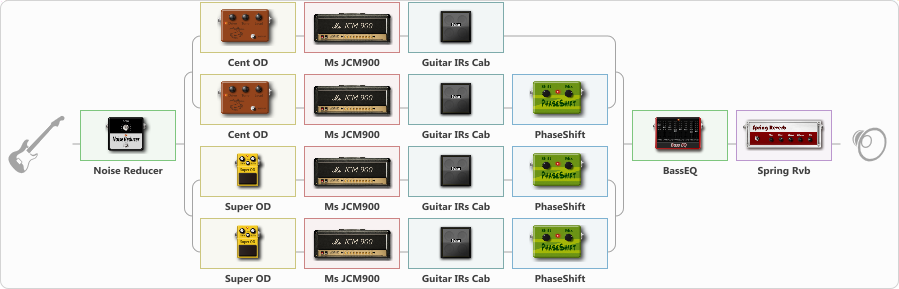# Working by may self

Discussion in 'ToneLib-GFX presets' started by Balaban, Mar 2, 2022.

1. Working by may self

Preset name: Djently cruching

Effects chain:Effect: "Noise Reducer" (Dynamics / Filter), active - "yes"
{
"Sens" = 80
"Mode" = Hard
}

Effect: "Splitter" (Dynamics / Filter)
{
"A-Bypass" = Off
"A-Pan" = 50
"A-Level" = 70
"B-Bypass" = Off
"B-Pan" = -50
"B-Level" = 70
"Width" = 0

'A' branch:
{

Effect: "Splitter" (Dynamics / Filter)
{
"A-Bypass" = Off
"A-Pan" = -65
"A-Level" = 55
"B-Bypass" = Off
"B-Pan" = -35
"B-Level" = 55
"Width" = 0

'A' branch:
{

Effect: "Cent OD" (Overdrive / Distortion), active - "yes"
{
"Drive" = 68
"Tone" = 42
"Level" = 59
}

Effect: "Ms JCM900" (Amp simulators), active - "yes"
{
"Gain" = 42
"Bass" = 17
"Middle" = 14
"Treble" = 36
"Presence" = 67
"Master" = 70
"Level (dB)" = -2
}

Effect: "Guitar IRs Cab" (Cabinets), active - "yes"
{
"Model" = Mesa Rectifier (4x12")
"Mic Position" = Middle
"Mic Distance" = Far
"Low Cut (Hz)" = 82
"Hi Cut (kHz)" = 8.0
"Mix" = 100
"Level (dB)" = 4
}
}
'B' branch:
{

Effect: "Cent OD" (Overdrive / Distortion), active - "yes"
{
"Drive" = 68
"Tone" = 42
"Level" = 59
}

Effect: "Ms JCM900" (Amp simulators), active - "yes"
{
"Gain" = 42
"Bass" = 17
"Middle" = 14
"Treble" = 36
"Presence" = 67
"Master" = 70
"Level (dB)" = -2
}

Effect: "Guitar IRs Cab" (Cabinets), active - "yes"
{
"Model" = Marshall JCM2000 (4x12")
"Mic Position" = Middle
"Mic Distance" = Middle
"Low Cut (Hz)" = 82
"Hi Cut (kHz)" = 8.0
"Mix" = 100
"Level (dB)" = 4
}

Effect: "PhaseShift" (Modulation / Sfx), active - "yes"
{
"Shift" = 36
"Mix" = 100
}
}
}
}
'B' branch:
{

Effect: "Splitter" (Dynamics / Filter)
{
"A-Bypass" = Off
"A-Pan" = 35
"A-Level" = 55
"B-Bypass" = Off
"B-Pan" = 65
"B-Level" = 55
"Width" = 0

'A' branch:
{

Effect: "Super OD" (Overdrive / Distortion), active - "yes"
{
"Drive" = 17
"Tone" = 72
"Level" = 91
}

Effect: "Ms JCM900" (Amp simulators), active - "yes"
{
"Gain" = 42
"Bass" = 17
"Middle" = 14
"Treble" = 36
"Presence" = 67
"Master" = 70
"Level (dB)" = -2
}

Effect: "Guitar IRs Cab" (Cabinets), active - "yes"
{
"Model" = Fender Deluxe Rev (1x12")
"Mic Position" = Edge
"Mic Distance" = Far
"Low Cut (Hz)" = 82
"Hi Cut (kHz)" = 8.0
"Mix" = 100
"Level (dB)" = 4
}

Effect: "PhaseShift" (Modulation / Sfx), active - "yes"
{
"Shift" = 54
"Mix" = 100
}
}
'B' branch:
{

Effect: "Super OD" (Overdrive / Distortion), active - "yes"
{
"Drive" = 20
"Tone" = 62
"Level" = 91
}

Effect: "Ms JCM900" (Amp simulators), active - "yes"
{
"Gain" = 42
"Bass" = 17
"Middle" = 14
"Treble" = 36
"Presence" = 67
"Master" = 70
"Level (dB)" = -2
}

Effect: "Guitar IRs Cab" (Cabinets), active - "yes"
{
"Model" = Mesa Rectifier (4x12")
"Mic Position" = Middle
"Mic Distance" = Near
"Low Cut (Hz)" = 82
"Hi Cut (kHz)" = 8.0
"Mix" = 100
"Level (dB)" = 4
}

Effect: "PhaseShift" (Modulation / Sfx), active - "yes"
{
"Shift" = 71
"Mix" = 100
}
}
}
}
}

Effect: "BassEQ" (Dynamics / Filter), active - "yes"
{
"50 Hz" = 9
"120 Hz" = 0
"400 Hz" = -2
"500 Hz" = -2
"800 Hz" = 3
"4.5 kHz" = 3
"10 kHz" = -13
"Level (dB)" = 0
}

Effect: "Spring Rvb" (Reverberation), active - "yes"
{
"Time" = 7.0
"PreDelay" = 0
"LoDamp" = 16
"HiDamp" = 30
"Mix" = 33
}

Note: You will need to download and install the ToneLib-GFX software to use the preset.

File size:
1.9 KB
Views:
2,368